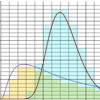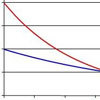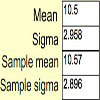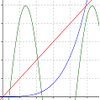Search by Topic

Filter by: Content type:
Age range:
Challenge level:

There are 17 NRICH Mathematical resources connected to Probability distributions, expectation and variance, you may find related items under Advanced Probability and Statistics.

Broad Topics > Advanced Probability and Statistics > Probability distributions, expectation and varianceAim High

Age 16 to 18 Challenge Level:

How do you choose your planting levels to minimise the total loss at harvest time?Age 16 to 18 Challenge Level:

Can you work out the means of these distributions using numerical methods?Time to Evolve 2

Age 16 to 18 Challenge Level:

How is the length of time between the birth of an animal and the birth of its great great ... great grandparent distributed?Stats Statements

Age 16 to 18 Challenge Level:

Are these statistical statements sometimes, always or never true? Or it is impossible to say?Into the Exponential Distribution

Age 16 to 18 Challenge Level:

Get into the exponential distribution through an exploration of its pdf.Random Inequalities

Age 16 to 18 Challenge Level:

Can you build a distribution with the maximum theoretical spread?Spinners

Age 16 to 18 Challenge Level:

How do scores on dice and factors of polynomials relate to each other?A Well-stirred Sample

Age 16 to 18 Challenge Level:

Typical survey sample sizes are about 1000 people. Why is this?Binomial or Not?

Age 16 to 18 Challenge Level:

Are these scenarios described by the binomial distribution?What Is a Random Variable, Really?

Age 16 to 18Binomial Conditions

Age 16 to 18 Challenge Level:

When is an experiment described by the binomial distribution? Why do we need both the condition about independence and the one about constant probability?Can You Find ... Random Variable Edition

Age 16 to 18 Challenge Level:

Can you create random variables satisfying certain conditions?Statistics - Maths of Real Life

Age 14 to 18 Challenge Level:

This pilot collection of resources is designed to introduce key statistical ideas and help students to deepen their understanding.Exponential Intersection

Age 16 to 18 Challenge Level:

Can the pdfs and cdfs of an exponential distribution intersect?Understanding Hypotheses

Age 14 to 18

This article explores the process of making and testing hypotheses.Data Matching

Age 14 to 18 Challenge Level:

Use your skill and judgement to match the sets of random data.Target Archery

Age 7 to 11 Challenge Level:

A simulation of target archery practice